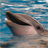## Mcgen3PhWaveSineIntp  - Generate 3-Phase Sine Wave   (doubts)

cancel
Showing results for
Did you mean:

## Mcgen3PhWaveSineIntp  - Generate 3-Phase Sine Wave   (doubts)

424 ViewsContributor II

Hello colleagues,

I'm a begginer using a microcontroller DSC MC56F8006, and i´m trying to create a code using the beans of Processor Expert. My goal is to create a PWM to control a 3 phase inverter, with phase shifting and with sine base frequency at 50HZ. Before i tried with code (using a look-up table) but i had a lot of troubles.

So, now i'm learning this function precisely, (Mcgen3PhWaveSineIntp) inserted in the Motor Control Bean's, and in combination with PWMMC Bean i can achieve my goal.

*

1) How can find the correspondent value to the variable   "PhaseIncrement" ?

2) The variable "DesiredFreq" will be in my case (50Hz)?

3)Which frequency should be on variable "FunctionCallFreq"?

4) Period of sine in my sine still being the value of 65535?

5) This two beans are enough for the procedure that i want to create? Or i dont even need the PWM bean?

Thanks in advance , I really appreciate any help that you can give me in this topic, because at this moment i'm stopped without know how to advance!

Regards,

Rui Pedro

**Notes:

PhaseIncrementis angle increment added to actual phase each function call [-].

DesiredFreq is desired frequency of generated waveform [Hz].

FunctionCallFreq is frequency of function calling [Hz].

Period is period of sine. In our case it is 65535.

Labels (1)
• ### General

4 Replies
147 ViewsContributor I

hello everyone,

i am new in NXP/FS device . microchip is ok. i want to design a sine wave inverter full bridge on HC8 mcu. anybuddy can help me about this?.

i know ALGO but in microchip device. some time before FREESCALE release a app notes on MC9S08SH4 mcu. can any buddy provide me for

learning purpose.

thanks to you

302 ViewsNXP TechSupport

Hi, Rui,

As you know that the 3-Phase Wave Generation algorithms is used to control an ACIM with VVVF algorithms. In general, we update the PWM duty cycle in ISR of PWM reload event.

Assume that you want to get 50HZ 3 phase signal, the PWM frequency is 8KHz, of course, the PWM reload interrupt frequency is 8KHz. In the case, the DesiredFreq is 50hz, the FunctionCallFreq is 8kHz, the point number for ONE the sine cycle is 8KHz/50Hz=160, the incremental angle is 360/160=2.25 degree.

This is the formula:

360 degree represents 65535, what is 2.25 degree? we use the formula 360:65535=2.25:x, the x=65535/(360/2.25)=65535/160=410, the PhaseIncrement is 410.

That is why of Eqn 4-9 formula in MotorControl.pdf doc.

You have to add the PhaseGeneration bean and PWN bean, the PhaseGeneration can calculate the phase voltage, the PWM beans can change the duty cycle of PWM based on the voltage from the PhaseGeneration bean.

I attach an example based on 56F8013, hope it can help you.

B R

Xiangjun rong

302 ViewsContributor II

Hi Xiangjun rong,

First of all thanks for your interest in my topic and help me. I understood your logic, in the sequence for get the values respecting the equation, but i still confused, like thinking in values for angles (degree). In the example of that equation 4-9 that you refereed they just talk about frequency:

Access frequency of function mcgen3PhWaveSineIntp based on interrupt frequency = 4kHz

• Desired generated sine wave frequency = 100Hz

• Parameter PhaseIncrement must be set to 1638 (100/4000*65535=1638)

For the same circumstances we will have different results. Btw, your logic is making me more sense.

Can i use that beans without receive in input feedback from the motor like (voltage and amplitude) ??

Sorry but i couldn´t see the attach that you did, can you repeat the please?

Thanks again,

Rui Mausinho

302 ViewsNXP TechSupport

Hi, Rui,

In the following condition:

Access frequency of function mcgen3PhWaveSineIntp based on interrupt frequency = 4kHz

• Desired generated sine wave frequency = 100Hz

• Parameter PhaseIncrement must be set to 1638 (100/4000*65535=1638)

The points number of ONE sine cycle is 4KHz/100Hz=40,

incremental angle is 360/40=9 degree.

This is the formula:

360 degree represents 65535, what is 9 degree? we use the formula 360:65535=9:x, the x=65535/(360/9)=65535/40=1638, the PhaseIncrement is 1638. Obviously, the result is the same. The the 3-Phase Wave Generation algorithms is used to control an ACIM with VVVF algorithms, the amplitude is proportional to the desired frequency, so i think the amplitude is a required parameter.